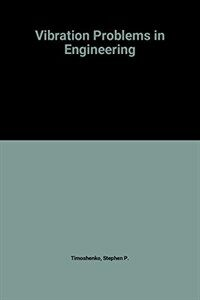> 상세정보

# 상세정보## Vibration problems in engineering 4th ed (23회 대출)

자료유형
단행본
개인저자
Timoshenko, Stephen, 1878-1972. Young, Donovan Harold, 1904-. Weaver, William, 1929-.
서명 / 저자사항
Vibration problems in engineering / [by] S. Timoshenko, D. H. Young [and] W. Weaver, Jr.
판사항
4th ed.
발행사항
New York :   Wiley,   1974.
형태사항
xiii, 521 p. : ill. ; 23 cm.
ISBN
0471873152
서지주기
Includes bibliographical references and index.
일반주제명
Vibration.
 000 00875camuu2200277 4500 001 000000618177 005 20130701171034 008 740329s1974 nyua b 001 0 eng 010 ▼a 74006191 020 ▼a 0471873152 040 ▼a DLC ▼c DLC ▼d FCU ▼d 211009 049 1 ▼l 421003257 ▼f 과개 050 0 ▼a TA355 ▼b .T55 1974 082 0 0 ▼a 620.3 ▼2 23 084 ▼a 620.3 ▼2 DDCK 090 ▼a 620.3 ▼b T585v4 100 1 ▼a Timoshenko, Stephen, ▼d 1878-1972. 245 1 0 ▼a Vibration problems in engineering / ▼c [by] S. Timoshenko, D. H. Young [and] W. Weaver, Jr. 250 ▼a 4th ed. 260 ▼a New York : ▼b Wiley, ▼c 1974. 300 ▼a xiii, 521 p. : ▼b ill. ; ▼c 23 cm. 504 ▼a Includes bibliographical references and index. 650 0 ▼a Vibration. 700 1 ▼a Young, Donovan Harold, ▼d 1904-. 700 1 ▼a Weaver, William, ▼d 1929-.

### 소장정보

No. 소장처 청구기호 등록번호 도서상태 반납예정일 예약 서비스
No. 1 소장처 청구기호 620.3 T585v4 등록번호 421003257 도서상태 대출가능 반납예정일 예약 서비스

### 컨텐츠정보

#### 목차

```
CONTENTS
CHAPTER 1 SYSTEMS WITH ONE DEGREE OF FREEDOM = 1
1.1 Free Harmonic Vibrations = 1
1.2 Rotational Vibrations = 13
1.3 Energy Method = 23
1.4 Rayleigh Method = 31
1.5 Beams and Shafts with Several Masses = 42
1.6 Forced Vibrations : Steady State = 48
1.7 Forced Vibrations : Transient State = 58
1.8 Free Vibrations with Viscous Damping = 63
1.9 Forced Vibrations with Viscous Damping = 72
1.10 Equivalent Viscous Damping = 81
1.11 General Periodic Disturbing Force = 89
1.12 Arbitrary Disturbing Force = 97
1.13 Arbitrary Support Motion = 110
1.14 Response Spectra = 120
1.15 Numerical Solutions for Response = 129
CHAPTER 2 SYSTEMS WITH NONLINEAR CHARACTERISTICS = 144
2.1 Examples of Nonlinear Systems = 144
2.2 Direct Integration for Velocity and Period = 159
2.4 Forced Nonlinear Vibrations = 177
2.5 Piecewise-Linear Systems = 186
2.6 Numerical Solutions for Nonlinear Systems = 203
CHAPTER 3 SYSTEMS WITH TWO DEGREES OF FREEDOM = 219
3.1 Examples of Two-Degree Systems = 219
3.2 Action Equations : Stiffness Coefficients = 225
3.3 Displacement Equations : Flexibility Coefficients = 231
3.4 Inertial and Gravitational Coupling = 239
3.5 Undamped Free Vibrations = 247
3.6 Undamped Forced Vibrations = 259
3.7 Free Vibrations with Viscous Damping = 267
3.8 Forced Vibrations with Viscous Damping = 272
CHAPTER 4 SYSTEMS WITH MULTIPLE DEGREES OF FREEDOM = 279
4.1 Introduction = 279
4.2 Frequencies and Mode Shapes for Undamped Systems = 280
4.3 Principal and Normal Coordinates = 296
4.4 Normal-Mode Response to Initial Conditions = 303
4.5 Normal-Mode Response to Applied Actions = 309
4.6 Normal-Mode Response to Support Motions = 317
4.7 Iteration Method for Frequencies and Mode Shapes = 327
4.8 Damping in Multi-Degree Systems = 342
4.9 Damped Response to Periodic Excitation = 346
4.10 Transient Response of Damped Systems = 351
4.11 Numerical Solutions for Transient Response = 355
CHAPTER 5 VIBRATIONS OF ELASTIC BODIES = 363
5.1 Introduction = 363
5.2 Free Longitudinal Vibrations of Prismatic Bars = 364
5.3 Forced Longitudinal Response of Prismatic Bars = 373
5.4 Normal-Mode Method for Prismatic Bars = 380
5.5 Prismatic Bar with a Mass or Spring at the End = 387
5.6 Bars Subjected to Longitudinal Support Motions = 395
5.7 Torsional Vibrations of Circular Shafts = 401
5.8 Transverse Vibrations of Stretched Wires = 408
5.9 Transverse Vibrations of Prismatic Beams = 415
5.10 Transverse Vibrations of a Simple Beam = 420
5.11 Vibrations of Beams with Other End Conditions = 424
5.12 Effects of Rotary Inertia and Shearing Deformations = 432
5.13 Forced Response of a Simple Beam = 435
5.14 Forced Response of Beams with Other End Conditions = 441
5.15 Beams Subjected to Support Motions = 443
5.16 Beams Traversed by Moving Loads = 448
5.17 Effect of Axial Force on Vibrations of Beams = 453
5.18 Beams on Elastic Supports or Elastic Foundations = 455
5.19 Ritz Method for Calculating Frequencies = 459
5.20 Vibrations of Nonprismatic Beams = 464
5.21 Coupled Flexural and Torsional Vibrations of Beams = 471
5.22 Vibrations of Circular Rings = 476
5.23 Transverse Vibrations of Membranes = 481
5.24 Transverse Vibrations of Plates = 492
APPENDIX : COMPUTER PROGRAMS = 503
A.1 Introduction = 503
A.2 Numerical Solutions for Linear Systems = 503
A.3 Numerical Solutions for Nonlinear Systems = 504
A.4 Iteration of Eigenvalues and Eigenvectors = 505
A.5 Numerical Solutions for Multi-Degree Systems = 505
INDEX = 515

```

### 관련분야 신착자료

#### 공학수학 문제풀이

Zill, Dennis G. (2022)

#### Design, fabrication and characterization of multifunctional nanomaterials

Thomas, Sabu (2021)

#### 통계적 품질관리

Montgomery, Douglas C. (2022)

#### 재료과학과 공학

Callister, William D. (2021)

#### Current research in neuroadaptive technology

Fairclough, Stephen H (2022)

장경욱 (2022)

#### Multiscale modeling approaches for composites

Chatzigeorgiou, George (2022)

김도영 (2022)

#### 재료공학개론

Shackelford, James F. (2022)

이지웅 (2021)

이정원 (2022)

송철기 (2022)

#### 공학수학

Zill, Dennis G. (2022)

유봉조 (2022)

이시중 (2022)

#### (Beer의) 재료역학

Beer, Ferdinand Pierre (2022)